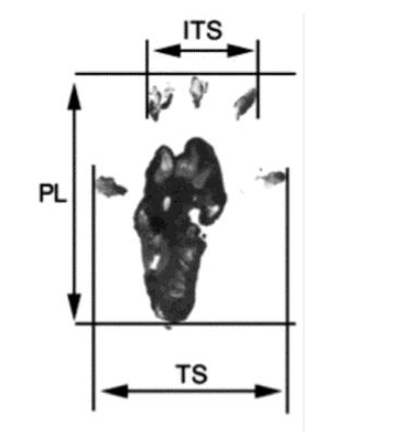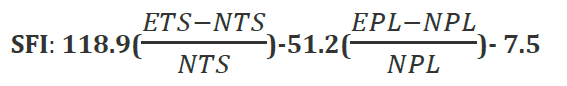مدل های رفتاری

# Behavioral Models _ motor function of rats

## (Sciatic functional index (SFI

Motor function was evaluated with the sciatic functional index (SFI) as previously described – each week after surgery, the animals’ pawprints were recorded, and two measurements were taken: (i) the print length (PL), corresponding to the distance from the heel to the third toe; and (ii) the toe spread (TS), corresponding to the distance from the first to fifth toe. Both measurements were taken from injured (E, for experimental) as well as noninjured (N, for normal) sides, and the SFI was calculated according to the following equation:The sciatic function index oscillates around zero for normal nerve function, and when it is equal to 2100 represents total loss of function of the nerve.

بستن